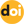The peer-reviewed scientific and technology journal. ISSN: 0204-3327

# Prediction of reliability of flow sensors of SHADR-32m heat carrierhttps://doi.org/10.26583/npe.2017.1.05

### UDC: 62-192:519.6

The complexity of technical systems, such as nuclear power plants, necessitates reliability assessments especially of the equipment that may affect the reactor operation safety. These assessments are also required for commercial reasons. In this context, of great importance are the tasks associated with investigation of regularities of the equipment and process parameter variations as well as development of methods and algorithms to obtain quantitative reliability indicators for the gradual (parametric) failures. The article considers these tasks with respect to the SHADR-32M flow rate sensors of RBMK-1000 reactor. Analysis was made of statistical data on periodic diagnostic measurements of the two defining performance parameters of SHADR-32M flow meters: the minimum value of the negative half-wave amplitude and standard deviation for the rotation period of the flow meter ball, which made it possible to develop a mathematical model of the sensor parametrical reliability. This flowmeter mathematical reliability model is a random process which is a superposition of a simple recovery process and a stochastic process with independent increments. A study of the mathematical model of the flow sensor operation reliability allowed us to obtain a closed form of the ratio of the flowmeter mean time to the crossing of a given border by each of the governing parameters and the probability of the flowmeter failure in the asymptotic setting without any assumptions about the laws of random variable distributions. The obtained results can be easily generalized for the case when the vector dimension of determining parameters is more than two without a significant complication of results. The use of the research results is illustrated by calculating quantitative parametric reliability indicators of the flow sensors.

1. Ostreykovskiy V.A. Salnikov N.L. Veroyatnostnoye prognozirovaniye rabotosposobnosti elementov YaEU [Probabilistic performance forecasting of nuclear power facility components]. Moscow. Energoatomizdat Publ., 1990. 416 p. (in Russian).
2. Druzhinin G.V. Nadezhnost avtomatizirovannykh system [Reliability of automated sysetms]. Moscow. Energiya Publ., 1977. 534 p. (in Russian).
3. Kapur S., Lamberson L. Nadezhnost i proektirovaniye system [Reliability in Engineering Design]. Trans. from Eng. Moscow. Mir Publ., 1980, 598 p. (in Russian).
4. Strelnikov V.P. Modeli otkazov mekhanicheskikh system [Mechanical system failure models]. Kiev. Znanie Publ., 1982. 22 p. (in Russian).
5. Pugachev V.S., Sinicyn I.N. Teoriya stokhasticheskikh system [Stochastic system theory]. Textbook. Moscow. Logos Publ., 2000, 1000 p. (in Russian).
6. Prokhorov Yu.V., Rozanov Yu.A. Teoriya veroyatnostey. Osnovnye ponyatiya. Predelnye teoremy. Sluchaynye protsessy [Theory of probability. Basic concepts. Limit theorems. Random processes]. Moscow. Nauka Publ., 1987, 400 p. (in Russian).
7. Korolyuk V.S., Portenko N.I., Skorokhod A.V., Turbin A.F. Spravochnik po teorii veroyatnostey i matematicheskoy statistike [Handbook on probability theory and mathematical statistics]. Kiev. Naukova Dumka Publ., 1978, 581 p. (in Russian).
8. Gikhman I.I., Skorokhod A.V. Stokhasticheskiye differencialnye uravneniya [Stochastic differential equations]. Kiev. Naukova Dumka Publ., 1968, 356 p. (in Russian).
9. Abramov O.V., Katuyeva Ya.V. Parallel algorithms for analysis and optimization of parametric reliability. Nadezhnost’, 2005, no. 4, pp. 19-26 (in Russian).
10. Augutis Yu., Alzbutas R., Matuzas V. Upravleniye nadezhnostyu sistemy izmereniya raskhoda teplonositelya ShADR432M v reaktore RBMK41500 [Reliability management of ShADR-32M coolant flow measurement system in RBMK-1500 reactor]. ISSN 0235 – 7208. Energetika [Power Engineering]. 2002, no. 4, pp. 27-32 (in Russian).
11. Pereguda A.I., Andreyev A.G. The asymptotic method for calculating durability indicators of products operating under shock loads. Nadezhnost’, 2007, v. 3, no. 22, pp. 31-39 (in Russian).
12. Pereguda A.I., Soborova I.A., Groshev A.I. Issues of assessing the reliability of products subject to discrete degradation. Izvestiya vuzov. Yadernaya energetika. 2000, no. 3, pp. 27-34 (in Russian).
13. Pereguda A.I., Andreyev A.G. Evaluation of reliability and durability of products subject to cyclical multidimensional impacts. Atomnaya energiya. 2007, v. 102, iss. 6, pp. 351-358 (in Russian).
14. Pereguda A.I., Soborova I.A. Nadezhnost i dolgovechnost. Modeli, pokazateli i metody ikh vychisleniya [Reliability and durability. Models, indicators and calculation methods]. Monograph. Obninsk: IATE Publ., 2006. 225 p. (in Russian).
15. Cox D., Smith. S. Teoriya vosstanovleniya [Renewal Theory] Trans. from Eng. Moscow. Sovetskoe radio Publ., 1967, 298 p. (in Russian).
16. Beichelt F., Franken P. Nadezhnost i tekhnicheskoye obsluzhivaniye. Matematicheskiy podkhod [Reliability and Maintenance. Mathematical Approach]. Trans. from Ger. Moscow. Radio i Svyaz’ Publ., 1988. 393 p. (in Russian).
17. Gikhman I.I., Skorokhod A.V. Teoriya sluchaynykh protsessov [Theory of random processes]. Vol. 2. Moscow. Nauka Publ., 1985. 640 p. (in Russian).
18. Ayvazyan S.A., Enyukov I.S., Meshalkin L.D. Prikladnaya statistika. Issledovaniye zavisimostey [Applied statistics. Research of dependencies]. Moscow. Finansy i Statistika Publ., 1985. 488 p. (in Russian).